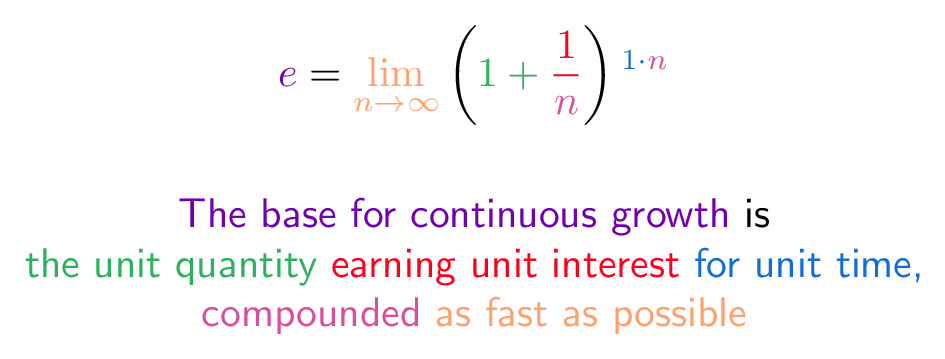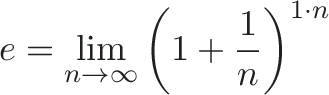# E (Compound Interest Definition)

## Colorized Definition\newcommand{\growth}{\color{c1}}
\newcommand{\unitQuantity}{\color{c2}}
\newcommand{\unitInterest}{\color{c3}}
\newcommand{\unitTime}{\color{c4}}
\newcommand{\perfectly}{\color{c5}}
\newcommand{\compounded}{\color{c6}}$\displaystyle{\growth e \plain = \perfectly \lim_{n\to\infty} \plain \left( \unitQuantity 1 + \unitInterest \frac{1}{\compounded n} \plain \right) \unitTime^{1 \cdot \compounded n} }$

\growth       The base for continuous growth
\plain        is
\
\unitQuantity the unit quantity
\unitInterest earning unit interest
\unitTime     for unit time,
\
\compounded   compounded
\perfectly    as fast as possible


## Plain English

• What is e? A constant (2.718...) representing continuous unit growth: the unit quantity (1.0), continuously growing the unit rate (100%), for unit time (1 period).

• Why's e special? All circles are the unit circle, scaled up. All continuously growing systems are e^{rt}, scaled to some rate and time.

• When should I use e? Use e^{rt} for things that change constantly (radioactive decay, populations). For growth based on discrete intervals (interest payments, combinatorics), (1 + rate)^{time} is a better model.

## Read More - [An Intuitive Guide To Exponential Functions & e](https://betterexplained.com/articles/an-intuitive-guide-to-exponential-functions-e/) - [Common Definitions of e (Colorized)](https://betterexplained.com/articles/definitions-of-e-colorized/) - [Q: Why is e special? (2.718..., not 2, 3.7 or another number?)](https://betterexplained.com/articles/q-why-is-e-special-2-718-not-other-number/)